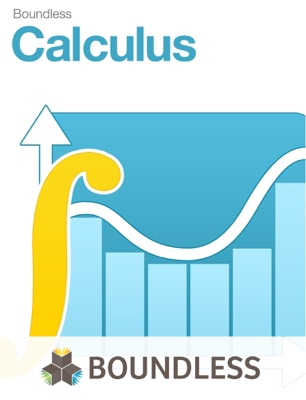### Calculus - Boundless

Relase Date: August 21, 2013
Publisher: Boundless
Category: Calculus

# Summary

Introduction to Calculus is a college-level, introductory textbook that covers the fascinating subject of Calculus. Boundless works with subject matter experts to select the best open educational resources available on the web, review the content for quality, and create introductory, college-level textbooks designed to meet the study needs of university students. This textbook covers: Building Blocks of Calculus -- Precalculus Review, Functions and Models, Limits Derivatives and Integrals -- Derivatives, Applications of Differentiation, Integrals, Applications of Integration Inverse Functions and Advanced Integration -- Inverse Functions: Exponential, Logarithmic, and Trigonometric Functions, Techniques of Integration, Further Applications of Integration Differential Equations, Parametric Equations, and Sequences and Series -- Differential Equations, Parametric Equations and Polar Coordinates, Infinite Sequences and Series Advanced Topics in Single-Variable Calculus and an Introduction to Multivariable Calculus -- Vectors and the Geometry of Space, Vector Functions, Partial Derivatives, Multiple Integrals, Vector Calculus, Second-Order Linear Equations